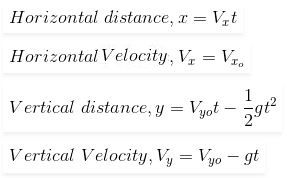The projection motion is one kind of motion. projectile is an entity thrown into the air or into space. A trajectory is a curved direction with which the projectile is moving. In a horizontal direction with constant velocity, the free-fall motion of any object is known as projectile motion.

Projectile Motion Formula  or trajectory formula is formulated asWhere,
Vx  = the velocity (along the x-axis)

Vxo = Initial velocity (along the x-axis)

Vy = velocity (along the y-axis)

Vyo = Initial velocity (along the y-axis)

g = acceleration due to gravity

t = Time taken

Equations related to the projectile motion is given as

Where
Vo = Initial Velocity
sin θ = Component along the y-axis
cos θ = component along the x-axis

The formula of projectile motion is used to calculate the velocity, distance and the time observed in the projectile motion of the object.

Projectile Motion Solved Example

Problem 1: Jhonson is standing on the top of the building and John is standing down. If Jhonson tosses a ball with velocity 30 m/s and at the angle of 70then at the time 3s what height will the ball reach?

Given:

Vyo = 30 m/s

Δ t = 3s

The Vertical velocity in y-direction is expressed as

Vy = Vyo sin 70o

Vy = 30 sin 70

Vy = 23.22 m/s